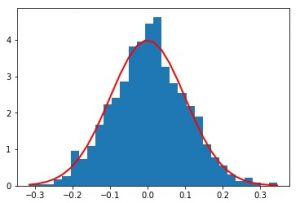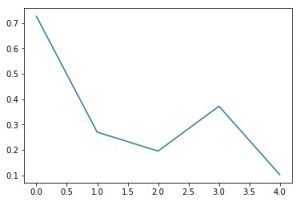# rand vs normal in Numpy.random in Python

• Last Updated : 17 Nov, 2020

In this article, we will look into the principal difference between the Numpy.random.rand() method and the Numpy.random.normal() method in detail.

• About random: For random we are taking .rand()
numpy.random.rand(d0, d1, …, dn) :
creates an array of specified shape and
fills it with random values.
Parameters :

```d0, d1, ..., dn : [int, optional]
Dimension of the returned array we require,

If no argument is given a single Python float
is returned.
```

Return :

```Array of defined shape, filled with random values.
```
• About normal: For random we are taking .normal()
numpy.random.normal(loc = 0.0, scale = 1.0, size = None) : creates an array of specified shape and fills it with random values which is actually a part of Normal(Gaussian)Distribution. This is Distribution is also known as Bell Curve because of its characteristics shape.
Parameters :

```loc   : [float or array_like]Mean of
the distribution.
scale : [float or array_like]Standard
Derivation of the distribution.
size  : [int or int tuples].
Output shape given as (m, n, k) then
m*n*k samples are drawn. If size is
None(by default), then a single value
is returned.
```

Return :

```Array of defined shape, filled with
random values following normal
distribution.
```
• Code 1 : Randomly constructing 1D array

 `# Python Program illustrating``# numpy.random.rand() method``  ` `import` `numpy as geek``  ` `# 1D Array``array ``=` `geek.random.rand(``5``)``print``(``"1D Array filled with random values : \n"``, array)`

Output :

```1D Array filled with random values :
[ 0.84503968  0.61570994  0.7619945   0.34994803  0.40113761]

```

Code 2 : Randomly constructing 1D array following Gaussian Distribution

 `# Python Program illustrating``# numpy.random.normal() method``  ` `import` `numpy as geek``  ` `# 1D Array``array ``=` `geek.random.normal(``0.0``, ``1.0``, ``5``)``print``(``"1D Array filled with random values "``      ``"as per gaussian distribution : \n"``, array)``# 3D array``array ``=` `geek.random.normal(``0.0``, ``1.0``, (``2``, ``1``, ``2``))``print``(``"\n\n3D Array filled with random values "``      ``"as per gaussian distribution : \n"``, array)`

Output :

```1D Array filled with random values as per gaussian distribution :
[-0.99013172 -1.52521808  0.37955684  0.57859283  1.34336863]

3D Array filled with random values as per gaussian distribution :
[[[-0.0320374   2.14977849]]

[[ 0.3789585   0.17692125]]]
```

Code3 : Python Program illustrating graphical representation of random vs normal in NumPy

 `# Python Program illustrating``# graphical representation of ``# numpy.random.normal() method``# numpy.random.rand() method``  ` `import` `numpy as geek``import` `matplotlib.pyplot as plot``  ` `# 1D Array as per Gaussian Distribution``mean ``=` `0` `std ``=` `0.1``array ``=` `geek.random.normal(``0``, ``0.1``, ``1000``)``print``(``"1D Array filled with random values "``      ``"as per gaussian distribution : \n"``, array);`` ` `# Source Code : ``# https://docs.scipy.org/doc/numpy-1.13.0/reference/``# generated/numpy-random-normal-1.py``count, bins, ignored ``=` `plot.hist(array, ``30``, normed``=``True``)``plot.plot(bins, ``1``/``(std ``*` `geek.sqrt(``2` `*` `geek.pi)) ``*``          ``geek.exp( ``-` `(bins ``-` `mean)``*``*``2` `/` `(``2` `*` `std``*``*``2``) ),``          ``linewidth``=``2``, color``=``'r'``)``plot.show()`` ` ` ` `# 1D Array constructed Randomly``random_array ``=` `geek.random.rand(``5``)``print``(``"1D Array filled with random values : \n"``, random_array)`` ` `plot.plot(random_array)``plot.show()`

Output :

```1D Array filled with random values as per gaussian distribution :
[ 0.12413355  0.01868444  0.08841698 ..., -0.01523021 -0.14621625
-0.09157214]1D Array filled with random values :
[ 0.72654409  0.26955422  0.19500427  0.37178803  0.10196284]```

Important :
In code 3, plot 1 clearly shows Gaussian Distribution as it is being created from the values generated through random.normal() method thus following Gaussian Distribution.
plot 2 doesn’t follow any distribution as it is being created from random values generated by random.rand() method.

Note :
Code 3 won’t run on online-ID. Please run them on your systems to explore the working.
.
This article is contributed by Mohit Gupta_OMG 😀. If you like GeeksforGeeks and would like to contribute, you can also write an article using contribute.geeksforgeeks.org or mail your article to contribute@geeksforgeeks.org. See your article appearing on the GeeksforGeeks main page and help other Geeks.

Please write comments if you find anything incorrect, or you want to share more information about the topic discussed above.

My Personal Notes arrow_drop_up